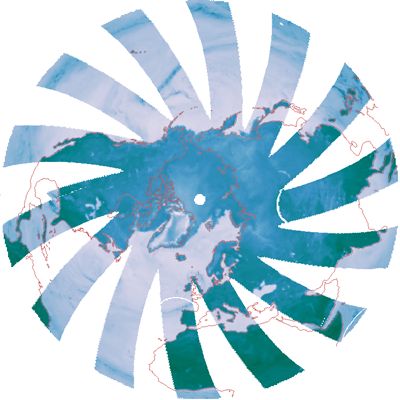# Creating a Transparent PNG Image

QUESTION: I have a 24-bit color image of my data. The missing data in the image is colored white. To use this data as a layer on my web page, I need to make a transparent PNG image. I'd like all the white pixels to be transparent. Is it possible to make a transparent PNG image in IDL?ANSWER: Yes, it is possible. Let's suppose we start with this 24-bit color image. We can read the file like this.

```   IDL> image = Read_PNG('maketransparentpng_1.png')    IDL> Help, image
IMAGE    BYTE = Array[3, 400, 400] ```

It is much easier to work with an image like this if it is frame-interleaved, rather that pixel-interleaved. We can use the Transpose command in IDL to turn this image into a frame-interleaved image, like this.

```   IDL> image = Transpose(image, [1,2,0])    IDL> Help, image
IMAGE    BYTE = Array[400, 400, 3] ```

The image looks like the figure below.This is the 24-bit image that serves as the starting point for making a transparent PNG image.

The missing color in this image is represented by the color white, which is a color triple with the value [255,255,255]. (If the missing color were black you would search instead for the color triple [0,0,0], and so forth.) The point is, you have to find these values by search in the red, green, and blue color planes of the image. This is much easier to do if you first deconstruct the image into these color planes, like this.

```    IDL> red = image[*,*,0]
IDL> grn = image[*,*,1]    IDL> blu = image[*,*,2] ```

Now, finding the desired color triple is easy. We use the Where command in IDL.

```   IDL> whiteIndices = Where((red eq 255) and (grn eq 255) and (blu eq 255), count)
```

An alpha channel is needed to create a transparent PNG file. An alpha channel is a fourth channel in a 24-bit image that is added to the usual red, green, and blue color channels. The values of the alpha channel range from 0 to 255 and represent a range of transparency from total transparency (0) to total opacity (255). In our example here, we want the white pixels to be totally transparent (have values of 0 in the alpha channel) and the rest of the pixels to be totally opaque (have values of 255 in the alpha channel). We create an alpha channel and make the white pixels transparent like this.

```    IDL> s = Size(image, /DIMENSIONS)
IDL> xsize = s & ysize = s    IDL> alpha = BytArr(xsize, ysize) + 255B
IDL> IF count GT 0 THEN alpha[whiteIndices] = 0 ```

We can now put the four image planes back together in a transparent image.

```    IDL> transparentImage = [ [[red]], [[grn]], [[blu]], [[alpha]] ]
IDL> Help, transparentImage          IMAGE    BYTE = Array[400, 400, 4]
```

To write a transparent PNG file, we require a pixel-interleaved image, rather than a frame-interleaved image. We again use the Transpose command in IDL.

```    IDL> transparentImage = Transpose(transparentImage, [2,0,1])
IDL> Help, transparentImage          IMAGE    BYTE = Array[4, 400, 400]
```

And now we simply write the PNG file.

`   IDL> Write_PNG, 'maketransparentpng_2.png', transparentImage `

You can download the transparent image if you like. If you wish to display a transparent image in IDL, you can follow the directions in this article. It is not always obvious that a transparent image is transparent. However if you open a transparent image in an application like Photoshop, the transparent pixels are represented by a checked pattern, like in the figure below.The transparent image as viewed in Photoshop.

## Update

On 5 November 2010 I introduced changes to cgImage that allow it to display a transparent image directly on a background image.

I also introduced the program cgTransparentImage that allows you to create a transparent image from either another image or from the contents of an IDL graphics window. You can specify the amount of transparency and a missing color or index (2D images) in several ways. You can also save the transparent image directly to a transparent PNG file.Version of IDL used to prepare this article: IDL 7.1.2Web Coyote's Guide to IDL Programming